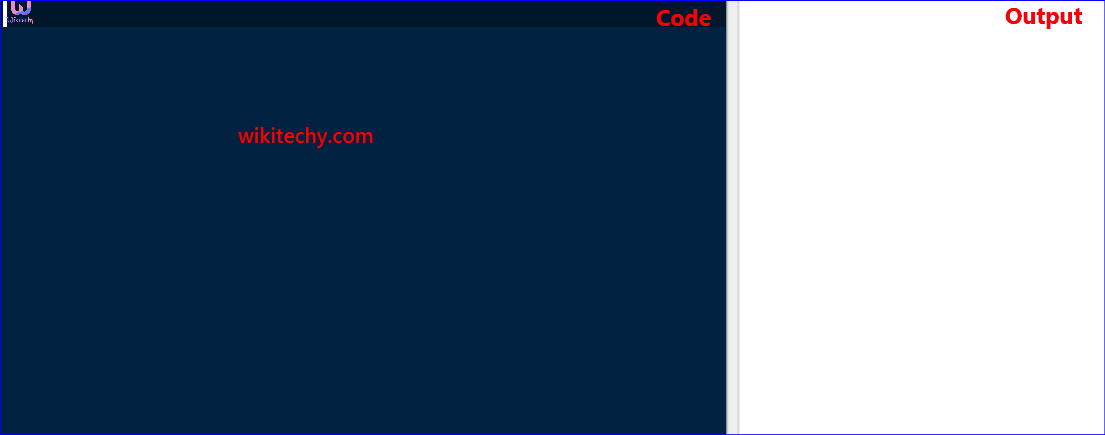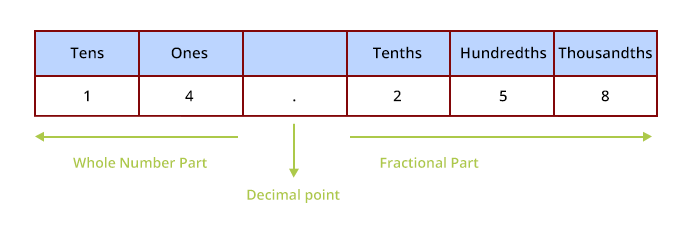# C program to Convert Decimal To Binary - Convert Decimal To Binary in CLearn C - C tutorial - c program to convert decimal to binary - C examples - C programs

## Decimal number

• A decimal number consists of a dot called decimal point.
• The decimal point separates the whole number part and the fractional part in a decimal number.
• The value of a digit according to the place of the digit in a number is known as the place value of that digit.Learn C - C tutorial - Decimal Number - C examples - C programs

## Binary number

• A number which uses only two symbols: 0 (zero) and 1 (one),is said to be the binary numbers.
• Any combination of 0 and 1 is binary number such as 1001, 101, 11111, 101010 etc.